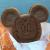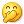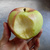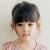怎么看待代码中使用没有意义的技巧？

```<?php

\$c = 1;
\$a = 1; \$b = 2;

empty(\$c) ? true : \$a = \$b;

// 直接写成

if (\$c) \$a = \$b; // 这样不就行了```

0`\$c && \$a = \$b;`00引用来自“fieldinrain”的评论`\$c && \$a = \$b;`

```if (!function_exists("pp")) {
function pp(\$data, \$exit = true, \$dump = false)
{
echo "<pre>";
\$dump || print_r(\$data);
\$dump && var_dump(\$data);
echo "</pre>";
\$exit && exit();
}
}```

```\$str = '';
for (\$i = 1; \$i < 3; \$i++) {
\$str .= ' ' . empty('d') ? '' : 'd';
}```